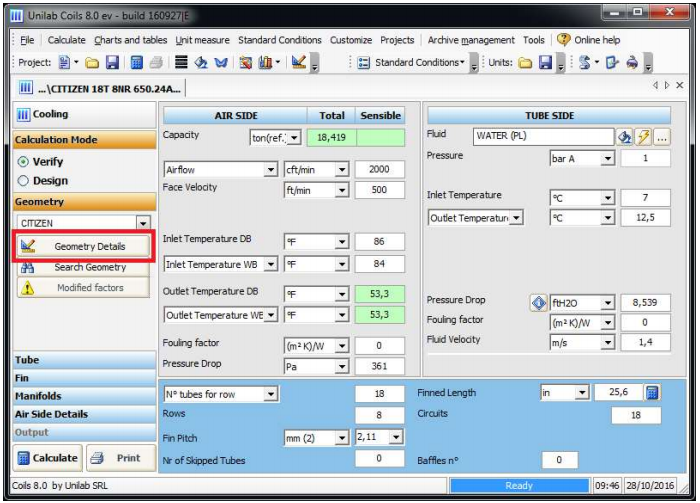## Factors details form### Descriptions of factors detailsAll the under factors can have a positive effect on the calculation only if obtained by laboratory test. They should not be used to trim performances of the coils without knowing the possible consequences

 Fin corrugation factor/slope: this parameter, defined as fin corrugation factor, represents the ratio (with the same air frontal velocity) between the exchange coefficient of a corrugated finned coil, and the corresponding exchange coefficient of a plain fin coil. This value is equal to 1 for plain fin. For further corrugation cases, the factors must be experimentally determined. Tube side coef. factor/slope: this parameter, defined as "coefficient factor inside tubes/slope", represents the ratio between the real exchange coefficient of internal tubes and the ideal one. This value is equal to 1 for cylindrical tubes with internal plain surface. For further cases, the factor must be experimentally determined Correction factor of friction factor/slope: this parameter, defined as "correction factor of friction factor/slope", represents the ratio between the real tubes internal side friction factor and the ideal one. This value is equal to 1 for cylindrical tubes with internal plain surface. For further cases, the factor must be experimentally determined. Friction factor air side/slope: this parameter, defined as "friction factor air side/slope", represents the ratio between the real tubes external side friction factor and the ideal one. This value is equal to 1 for plain fins. For further cases, the factor must be experimentally determined. “Slope” factors the coefficient of proportionality in logarithmic coordinates between the item at issue (fin etc…) and the related fluid speed. This parameter enables the user to find the correction factor in function of speed. Red/Inc Surface factor: this parameter, defined as the surface reduction/increase factor, is a nondimensional parameter which considers the possible calculation differences of the exchange surface. This factor permits to analyze those cases in which the geometry parameters are not constant, such as differentiated spacing coils (typical for direct expansion coils at very low temperature) or differentiated fin height coils. Reduction Area Pass. Tube: this parameter, defined as "tube passage reduction area", permits to consider possible elements which may reduce the fluid passage area. The value goes from 0 to 1. Latent air friction factor this factor applies specifically to calculations with dehumidification. In this type of application the fin is covered by water, which makes an increment on the air side pressure drops, calculated by a specific equation. This factor lets you trim the behavior of this equation. Tube internal surface ratio this parameter applies to grooved tubes and it lets you specify the ratio of the internal surface calculated like (Internal Grooved Surface / Internal Smooth Surface). This parameter is very important when the controlling resistance is tubes side. For a detailed explanation, please see the FAQ called “Considerations about grooved tubes” at the end of the manual. Security factor this parameter affects the entire calculation, and lets you increase or decrease the performance of the coil. It’s intended to be a factor to gain some security margin. Sensible factor this parameter affects the sensible capacity of the coil. The standard value is 0.6 and it is not proportional.

### What happens when the factors details are changedPlease don’t change the slope factors.

Fin corrugation factor

The default value is 1.

If the value increases / decreases, the coefficient of partial exchange air side increases / decreases.

Tube side coef. factor

The default value is 1.

If the value increases / decreases, the coefficient of partial exchange inside the tube increases / decreases.

Correction factor of friction factor

The default value is 1.

If the value increases / decreases, the inner tube side pressure drop increases / decreases.

Friction factor air side

The default value is 1.

If the value increases / decreases, the air side pressure loss  increases / decreases.

Red/Inc Surface factor

The default value is 1.

If the value increases / decreases, the heat exchange surface in the calculation increases / decreases and so the power increases / decreases.

Reduction Area Pass. Tube:

The default value is 1.

If the value increases / decreases, the passage area of the pipe decreases / increases.

Latent air friction factor

The default value is 1.

If the value increases / decreases, the load losses relating to condensed increases / decreases (you have to apply only to cooling coils of air)

Tube internal surface ratio

The default value is 1.

If the value increases / decreases, the tube internal exchange surface increases / decreases.

Security factor

The default value is 1.

If the value increases / decreases, the capacity increases / decreases.

Sensible factor

The default value is 0,6.

If the value increases / decreases, the sensitive capacity decreases / increases (you have to apply only to cooling coils of air).